International
Tables for
Crystallography
Volume D
Physical properties of crystals
Edited by A. Authier

International Tables for Crystallography (2006). Vol. D, ch. 1.1, pp. 5-6

## Section 1.1.2.3. Orthonormal frames of coordinates – rotation matrix

A. Authiera*

aInstitut de Minéralogie et de la Physique des Milieux Condensés, Bâtiment 7, 140 rue de Lourmel, 75015 Paris, France
Correspondence e-mail: aauthier@wanadoo.fr

#### 1.1.2.3. Orthonormal frames of coordinates – rotation matrix

| top | pdf |

An orthonormal coordinate frame is characterized by the fact that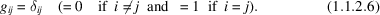One deduces from this that the scalar product is written simply as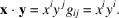Let us consider a change of basis between two orthonormal systems of coordinates: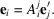Multiplying the two sides of this relation by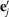, it follows that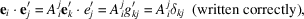which can also be written, if one notes that variance is not apparent in an orthonormal frame of coordinates and that the position of indices is therefore not important, as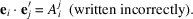The matrix coefficients,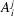, are the direction cosines ofwith respect to thebasis vectors. Similarly, we have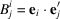so that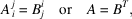where T indicates transpose. It follows that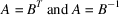so that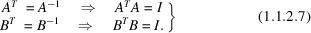The matrices A and B are unitary matrices or matrices of rotation and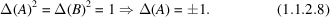If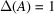the senses of the axes are not changed – proper rotation. If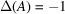the senses of the axes are changed – improper rotation. (The right hand is transformed into a left hand.)

One can write for the coefficients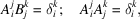giving six relations between the nine coefficients. There are thus three independent coefficients of thematrix A.